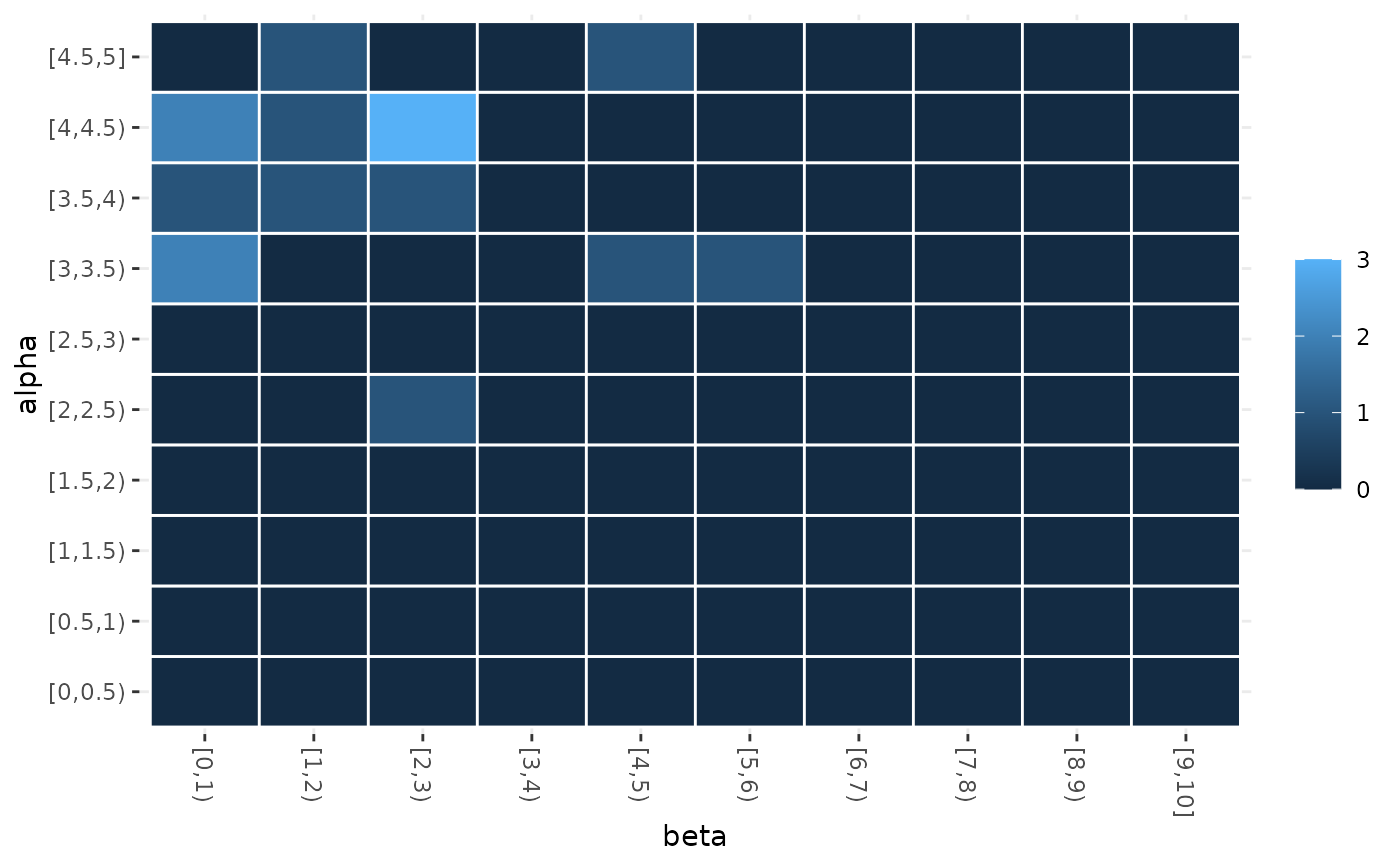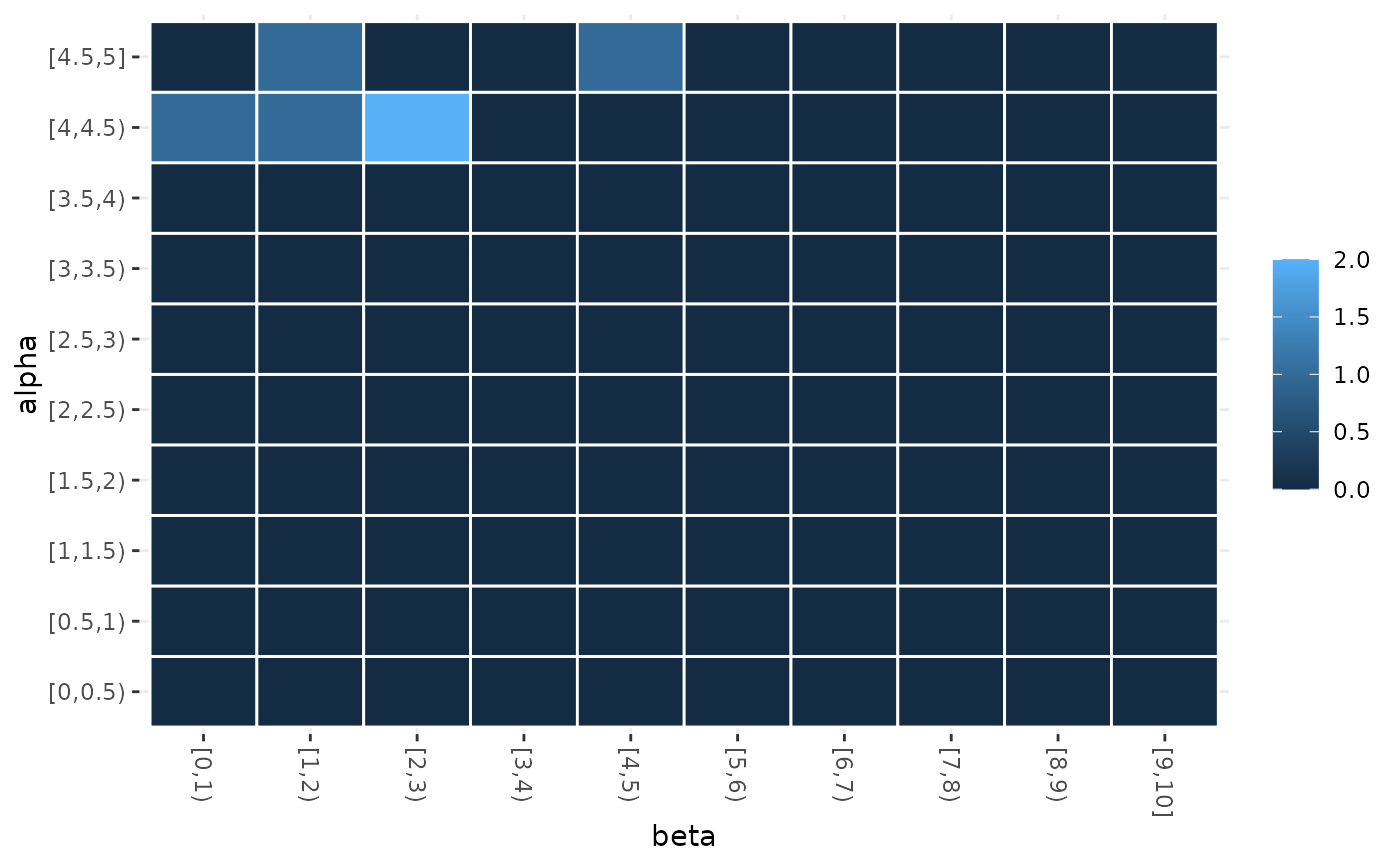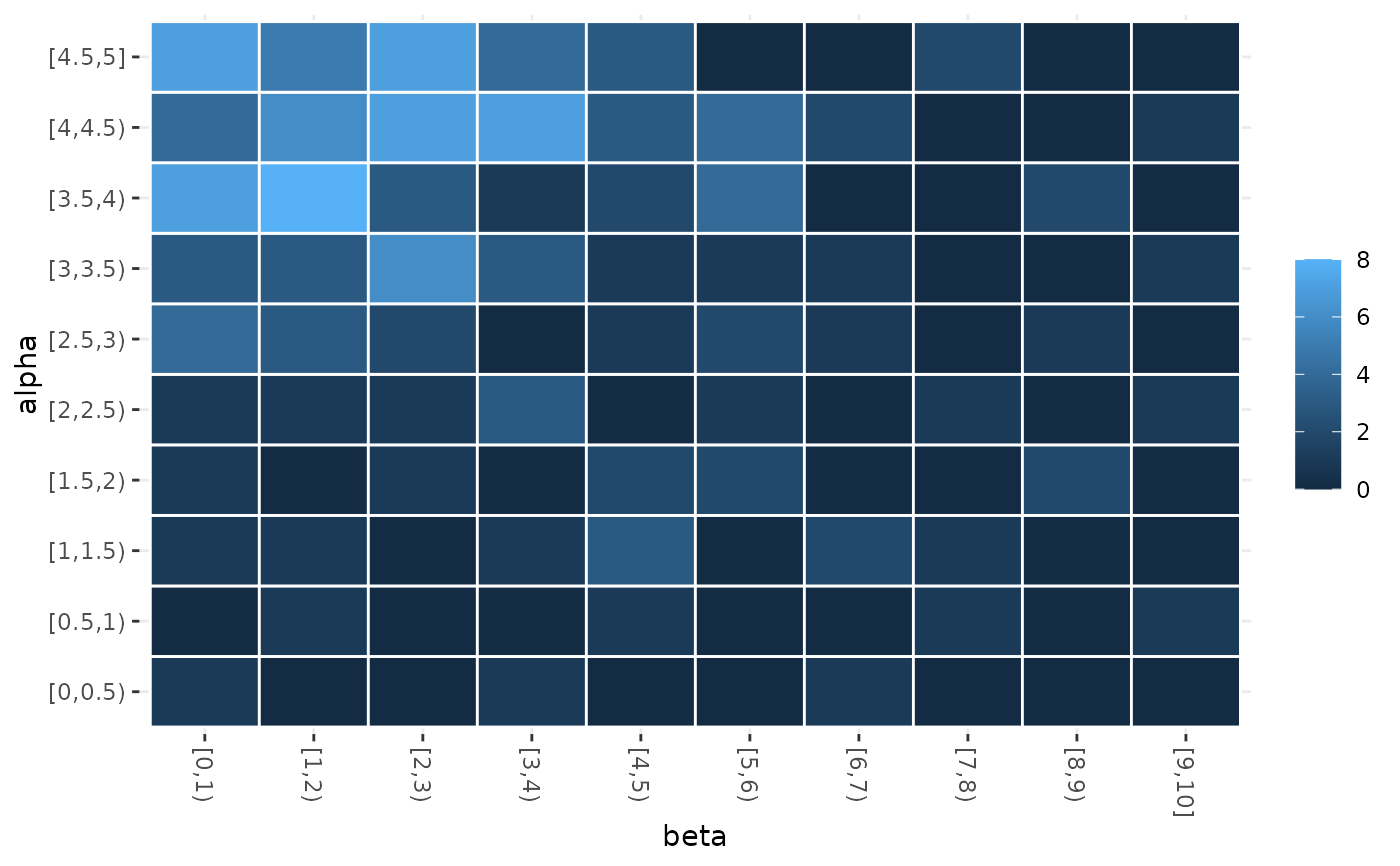Heatmap that displays the frequency of sampling values of two parameters.

sampling_heatmap(
irace_results,
param_names,
sizes = c(0, 0),
iterations = NULL,
only_elite = TRUE,
filename = NULL
)

## Arguments

irace_results

The data generated when loading the .Rdata file created by irace (or the filename of that file).

param_names

(character()) Parameters to be included in the plot. Example: c("algorithm","alpha","rho","q0","rasrank").

sizes

Numeric vector that indicated the number of intervals to be considered for numerical parameters. This argument is positional with respect to param_names. By default, numerical parameters are displayed using 10 intervals. (example sizes = c(0,10))

iterations

Numeric vector, iteration number that should be included in the plot (example: iterations = c(1,4,5))

only_elite

logical (default TRUE), only print elite configurations.

filename

(character(1)) File name to save the plot, for example "~/path/example/filename.png".

## Value

sampling heat map plot

## Examples

iraceResults <- read_logfile(system.file(package="irace", "exdata",
"irace-acotsp.Rdata", mustWork = TRUE))
sampling_heatmap(iraceResults, param_names=c("beta", "alpha"))sampling_heatmap(iraceResults, param_names=c("beta", "alpha"), iterations = c(3,4))sampling_heatmap(iraceResults, param_names=c("beta", "alpha"), only_elite = FALSE)